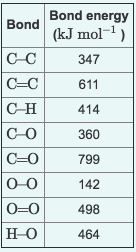# Problem: Use the table to answer questions about bond energies.a. Calculate the bond energy for breaking all the bonds in a mole of methane, CH4. Express your answer numerically in kJ/mole.Change in Heat CH4 ?=kJ/molb. Calculate the bond energy for breaking all the bonds in a mole of O2 molecules? Express your answer numerically in kJ/mole.Change in Heat O2 ? =kJ/molc. Calculate the bond energy for forming all the bonds in a mole of water molecules, H2O Express your answer numerically in kJ/mole.Change in Heat H2O =? kJ/mold. Calculate the bond energy for forming all the bonds in a mole of carbon dioxide, .Express your answer numerically in kJ/mole.Change in Heat CO2 =? kJ/mol

###### Problem Details

a. Calculate the bond energy for breaking all the bonds in a mole of methane, CH4. Express your answer numerically in kJ/mole.

Change in Heat CH4 ?=kJ/mol

b. Calculate the bond energy for breaking all the bonds in a mole of O2 molecules? Express your answer numerically in kJ/mole.

Change in Heat O2 ? =kJ/mol

c. Calculate the bond energy for forming all the bonds in a mole of water molecules, H2O Express your answer numerically in kJ/mole.

Change in Heat H2O =? kJ/mol

d. Calculate the bond energy for forming all the bonds in a mole of carbon dioxide, .Express your answer numerically in kJ/mole.

Change in Heat CO2 =? kJ/mol